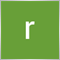problem to convert tick to double16

Hello,  i'm creating a volume at price indicator.

but  after receiving the value I don't know how to convert ..

MqlTick tick_array[];

double volume1m =0;

/////////the problem is here:
if (tick_array.last > 0)
{
volume1m += tick_array.last;
}
Print (volume1m);

/////I get similar value to this:         4.654716935610645e-212

could someone help me ?151135

really koh:

Hello,  i'm creating a volume at price indicator.

but  after receiving the value I don't know how to convert ..

MqlTick tick_array[];

double volume1m ;

the problem is here:

volume1m += tick_array.volume;

Print (volume1m );

I get similar value to this:         4.654716935610645e-212

could someone help me ?

You did not initialize volume1m to 0 prior to adding some value to it16

You did not initialize volume1m to 0 prior to adding some value to it

but, its works, its only an example, the problem is to convert like TimeToString, i need something like TickToDouble19815

really koh: , i need something like TickToDouble

Makes no sense. tick_array.last and volume1m  are already doubles.16

I found the problem!

the problem is:

IF  tick_array.volume is 0  and the variable took it

if i use

if (volume1m > 0)
{
Print();  // it enters and returns encrypted value
}

volume1m should be 0, becouse it took the value 0 from tick_array.. BUT NO :(
So i change to >

if (tick_array.last > 0)
{
volume1m =  tick_array[uz].last;
}

if (volume1m > 0)
{
Print();
}

and solved my problem

thank you!